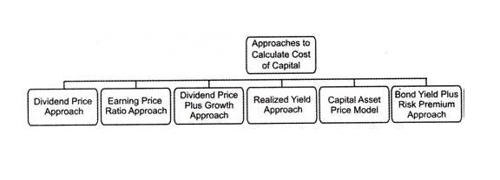An ordinary share is the sum of money raised by a corporate from private and public sources through the issue of its common shares. Cost of Ordinary Shares is the minimum rate of return which a company must earn to convince investors to invest in the company’s common stock at its current market price.

Approaches of Calculating Cost of Ordinary Shares or Equity Shares

Cost of equity is an important input in different stock valuation models such as the dividend discount model, H- model, residual income model, and free cash flow to equity model. It is also used in the calculation of the weighted average cost of capital. Different approaches to calculating the cost of ordinary shares or common stock or equity shares are as follows:

(1) Earning Yield Approach

This approach tells that we should not co-relate dividends per share with a market value per share but we should use total earning and try to co-relate it with the market value of shares. When the earning per share or net income after tax is given and there is no information regarding the dividend of an ordinary share, the cost of ordinary share can be calculated on the basis of earning and the market price of shares as shown below:

Ke = Earning per share/Market price per share or, EPS/MPS or, EPS/NP

(2) Dividend Yield Approach

According to the dividend price approach, we can calculate the cost of capital just dividing dividends per share with a market value of per share. When the dividend per share or total equity dividend is given and there is no information regarding the growth rate, the cost of equity share can be calculated on the basis of dividend and market price of shares as below:
Ke = Dividend per share/Market price per share or, DPS/MPS or, D/NP(3) Dividend Yield Plus Growth Rate Approach

This approach is an improvement in the dividend price approach for calculating the cost of capital. This is the most popular method of calculating the cost of equity shares. Under this method, the cost of equity share is determined on the basis of the following information:

(i) Current Dividend(Do): The dividend which has been recently paid and referred to as last year’s dividend, previous dividend, past dividends, etc.

(ii) Growth Rate In Dividend (g): The rate at which the annual earnings or dividend is increasing. When the growth rate is not given, it can be computed on the basis of past dividends or earning.

(iii) Expected Dividend (D1): The dividend which will be paid to the shareholders in the recent future is called the expected dividend. It is also referred to as the next dividend, coming dividend, future dividend, subsequent dividend, etc. On the basis of current dividend and past growth rate, the expected dividend can be determined as below:
Expected dividend = Current dividend(1+ growth rate) or, D1 = Do(1+g)

(iv) Net proceeds or Net Market Price (NP): The net selling price of share after deducting all kinds of issuing expense is known as net proceeds. The expenses incurred in the process of issuing the shares is called a flotation cost. Flotation cost includes brokerage fee, commission, and other publicity and administrative expenses. Net proceeds are determined as follows:

Net proceed (NP) = Gross selling price – Flotation cost = Gross selling price(1-Flotation cost) = Po(1-f)

On the basis of the above information, the cost of equity shares can be calculated as given below by using the dividend yield plus growth rate approach: Ke = (D1/NP) + g = Dividend yield plus Growth rate